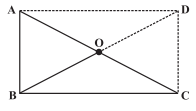# NCERT Solutions for Class 8 maths Chapter 3 Exercise 3.4

In this page we have NCERT Solutions for Class 8 maths Chapter 3 Exercise 3.4 .This exercise has questions about Special Types of Parallelogram like square, kite,rectangle and rhombus . Hope you like them and do not forget to like , social share and comment at the end of the page.

## NCERT Solutions for Class 8 maths Chapter 3 Exercise 3.4

Question 1
State whether True or False.
(a) All rectangles are squares.
(b) All rhombuses are parallelograms.
(c) All squares are rhombuses and also rectangles.
(d) All squares are not parallelograms.
(e) All kites are rhombuses.
(f) All rhombuses are kites.
(g) All parallelograms are trapeziums.
(h) All squares are trapeziums.
1. False:  All squares are rectangles but all rectangles are not squares
2. True
3. True
4. False
5. False
6. True
7. True
8. True

Question 2
Identify all the quadrilaterals that have
(a) Four sides of equal length
(b) Four right angles
(i)Square and Rhombus
(ii) Squares, rectangles

Question 3
Explain how a square is.
(ii) A parallelogram
(iii) A rhombus
(iv) A rectangle
(i) Square is quadrilateral as it has four sides
(ii) A square is parallelogram as opposite’s sides are parallel to each other
(iii) A square is rhombus as it has four sides equal
(iv)  A square is rectangles since each interior angles is 900

Question 4
(i) Bisect each other
(ii)are perpendicular bisectors of each other?
(iii)are equal
(i) The diagonals of parallelogram,square,rectangles,rhombus bisect each other
(ii)The diagonals of rhombus and square are perpendicular bisector of each other

Question 5
Explain why a rectangle is a convex quadrilateral.
A rectangles has two diagonals and both are in the interior, hence it is convex quadrilaterals

Question 6
ABC is a right-angled triangle and O is the mid point of the side opposite to the right angle. Explain why O is equidistant from A, B and C. (The dotted lines are drawn additionally to help you)AD and DC are parallel to BC and AB respectively
Also Angle ABC is 90° , So all the angles are equal and 90°
Hence it is a rectangle with equal angle and equal oppossite sides
Now in a rectangle, Diagonal Bisect each other and equal
So AO=OB=OC =OD
Hence O is equidistant from A, B and C

## Summary

1. NCERT Solutions for Class 8 Maths Chapter 3 Exercise 3.4 has been prepared by Expert with utmost care. If you find any mistake.Please do provide feedback on mail. You can download the solutions as PDF in the below Link also
2. This chapter 3 has total 4 Exercise 3.1 ,3.2,3.3 and 3.4. This is the Last exercise in the chapter.You can explore previous exercise of this chapter by clicking the link below